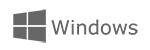```CycloAgentWith CycloAgent, you can manage software updates (excluding Cyclo 100 Series), synchronise your recordings to MioShare and also back up your Cyclo data. Before downloading CycloAgent you will need a MioShare account. Go to MIOSHARE and select Sign Up. Once your account has been verified, CycloAgent can be downloaded and you can use your MioShare credentials to log in. For more information about CycloAgent click HERE
.buttons{
display:inline-block;
}

function MM_swapImgRestore() { //v3.0
var i,x,a=document.MM_sr; for(i=0;a&&i<a.length&&(x=a[i])&&x.oSrc;i++) x.src=x.oSrc;
}
var d=document; if(d.images){ if(!d.MM_p) d.MM_p=new Array();
if (a[i].indexOf("#")!=0){ d.MM_p[j]=new Image; d.MM_p[j++].src=a[i];}}
}

function MM_findObj(n, d) { //v4.01
var p,i,x;  if(!d) d=document; if((p=n.indexOf("?"))>0&&parent.frames.length) {
d=parent.frames[n.substring(p+1)].document; n=n.substring(0,p);}
if(!(x=d[n])&&d.all) x=d.all[n]; for (i=0;!x&&i<d.forms.length;i++) x=d.forms[i][n];
for(i=0;!x&&d.layers&&i<d.layers.length;i++) x=MM_findObj(n,d.layers[i].document);
if(!x && d.getElementById) x=d.getElementById(n); return x;
}

function MM_swapImage() { //v3.0
var i,j=0,x,a=MM_swapImage.arguments; document.MM_sr=new Array; for(i=0;i<(a.length-2);i+=3)
if ((x=MM_findObj(a[i]))!=null){document.MM_sr[j++]=x; if(!x.oSrc) x.oSrc=x.src; x.src=a[i+2];}
}function MM_swapImgRestore() { //v3.0
var i,x,a=document.MM_sr; for(i=0;a&&i<a.length&&(x=a[i])&&x.oSrc;i++) x.src=x.oSrc;
}
var d=document; if(d.images){ if(!d.MM_p) d.MM_p=new Array();
if (a[i].indexOf("#")!=0){ d.MM_p[j]=new Image; d.MM_p[j++].src=a[i];}}
}

function MM_findObj(n, d) { //v4.01
var p,i,x;  if(!d) d=document; if((p=n.indexOf("?"))>0&&parent.frames.length) {
d=parent.frames[n.substring(p+1)].document; n=n.substring(0,p);}
if(!(x=d[n])&&d.all) x=d.all[n]; for (i=0;!x&&i<d.forms.length;i++) x=d.forms[i][n];
for(i=0;!x&&d.layers&&i<d.layers.length;i++) x=MM_findObj(n,d.layers[i].document);
if(!x && d.getElementById) x=d.getElementById(n); return x;
}

function MM_swapImage() { //v3.0
var i,j=0,x,a=MM_swapImage.arguments; document.MM_sr=new Array; for(i=0;i<(a.length-2);i+=3)
if ((x=MM_findObj(a[i]))!=null){document.MM_sr[j++]=x; if(!x.oSrc) x.oSrc=x.src; x.src=a[i+2];}
}```